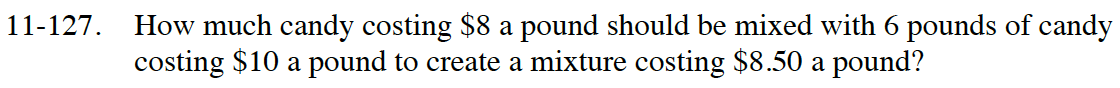Home > CC4 > Chapter 11 > Lesson 11.2.6 > Problem11-127

11-127.

How much candy costing $8 a pound should be mixed with 6 pounds of candy costing$10 a pound to create a mixture costing $8.50 a pound? Homework Help ✎Write an expression for the total cost. Write another expression for the total pounds. Define your variable: x = the number of pounds of$8

cost: 8x + 10(6)
total pounds: x + 6

Use the hint and expressions above to write your equation and solve for x.